Courses

# Past Year Questions: Working Stress & Limit State Method Notes | EduRev

## Civil Engineering (CE) : Past Year Questions: Working Stress & Limit State Method Notes | EduRev

The document Past Year Questions: Working Stress & Limit State Method Notes | EduRev is a part of the Civil Engineering (CE) Course RCC & Prestressed Concrete.
All you need of Civil Engineering (CE) at this link: Civil Engineering (CE)

Q.1 When a specimen of M25 concrete is loaded to a stress level of 12.5 MPa, a strain of 500 x 10-6 is recorded. If this load is allowed to stand for a long time, the strain increases to 1000 x 10-6. In accordance with the provisions of IS:456-2000, considering the long-term effects, the effective modulus of elasticity of the concrete (in MPa) is_______ .    [2019 : 2 Marks, Set-II]
Solution:

Initial strain = 500 x 10-6
stress = 12.5 N/mm2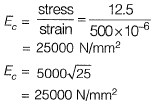Total strain after long time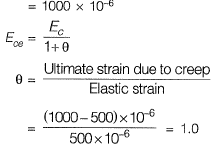Effective modulus of elasticity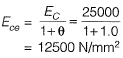Q.2 The characteristic compressive strength of concrete required in a project is 25 MPa and the standard deviation in the observed compressive strength expected at site is 4 MPa. The average compressive strength of cubes tested at different water-cement (w/c) ratios using the same material as is used for the project is given in the table.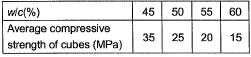The water-cement ratio (in percent, round off to the lower integer) to be used in the mix is______.    [2019 : 1 Mark, Set-II]
Solution:
Target mean strength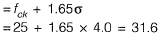Water content required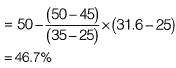Say 46%(round off to the lower integer)

Q.3 In the reinforced beam section shown in the figure (not drawn to scale), the nominal cover provided at the bottom of the beam as per IS 456-2000, is    [2019 : 1 Mark, Set-I]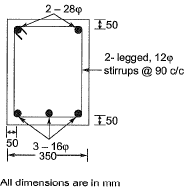(a) 30 mm
(b) 50 mm
(c) 42 mm
(d) 36 mm
Ans.
(A)
Solution:
Nominal cover = Effective cover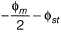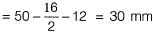Q.4 As per IS 456 : 2000, the minimum percentage of tension reinforcement (up to two decimal places) required in reinforced-concrete beams of rectangular cross-section (considering effective depth in the calculation of area) using Fe500 grade steel is _______.    [2018 : 1 Mark, Set-II]
Solution:

From clause 26.5.1.1 of IS 456 : 2000, we know minimum reinforcement is given by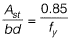∴ Min. % of steel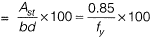For Fe500, min. % of steel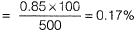Q.5 The frequency distribution of the compressive strength of 20 concrete cube specimen is given in the table.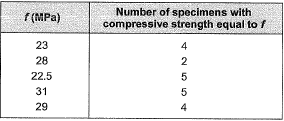If μ is the mean strength of the specimens and o is the standard deviation, the number of specimens (out of 20) with compressive strength less than μ - 3σ is _______ .    [2018 : 1 Mark, Set-I]
Solution:

Average strength,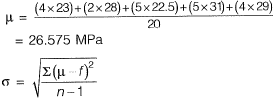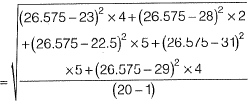= 3.7
Now, μ - 3σ = 26.575 - 3 x 3.7 = 15.48
Thus, no specimen is having compressive strength less than μ - 3σ.
No. of specimen = 0.

Q.6 The deformation in concrete due to sustained loading is    [2018 : 1 Mark, Set-I]
(a) creep
(b) hydration
(c) segregation
(d) shrinkage
Ans.
(A)
Solution:

Q.7 Let the characteristic strength be defined as that value, below which not more than 50% of the results are expected to fail. Assuming a standard deviation of 4 MPa, the target mean strength (in MPa) to be considered in the mix design of a M25 concrete would be    [2017 : 1 Mark, Set-II]
(a) 18.42
(b) 21.00
(c) 25.00
(d) 31.58
Ans.
(C)
Solution: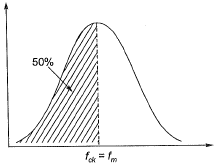If fck is the value below which not more than 50% of test results are expected then,
fm = fck
[Mean strength = Characteristics strength]
So target mean strength of concrete to be considered in design mix.
= Mean strength
fm = fck  = 25 MPa

Q.8 According to IS 456-2000, which one of the following statements about the depth of neutral axis xμbal for a balanced reinforced concrete section is correct?    [2017 : 1 Mark, Set-I]
(a) xμbal depends on the grade of concrete only
(b) xμbal  depends on the grade of steel only
(c) xμbal depends on both the grade of concrete and grade of steel
(d) xμbal does not depend on the grade of concrete and grade of steel
Ans.
(B)
Solution: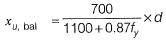So it depends upon grade of steel only.

Q.9 For M25 concrete with creep coefficient of 1.5, the long-term static modulus of elasticity (expressed in MPa) as per the provisions of IS:456-2000 IS: _______    [2016 : 2 Marks, Set-I]
Solution:

Long term static modulus of elasticity,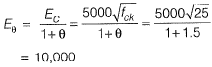Q.10 According to the concept of Limit State Design as per IS 456 : 2000, the probability of failure of a structure is _________.    [2015 : 2 Marks, Set-II]
Solution:

The structure may fail when,
(ii) the strength is less than the characteristic strength
(iii) both the load exceeds the design load and strength is less than the characteristic strength. The probability of load exceeding design load = 5%
(iv) The probability of strength less than characteristic strength = 5%.
The probability of failure is
= 0.05 x 0.95 + 0.05 x 0.95 + 0.05 x 0.05
= 0.0975

Q.11 Consider the singly reinforced beam section given below (left figure). The stress block parameters for the cross-section from IS 456 : 2000 are also given below (right figure). The moment of resistance for the given section by the limit state method is________kN-m.    [2015 : 2 Marks, Set-I]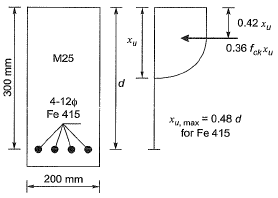Solution: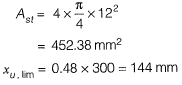∴ C = T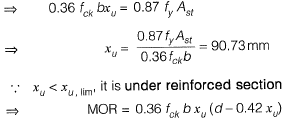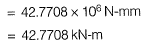Q.12 Consider ihe following statements for air-entrained concrete:
(i) Air-entrainment reduces the water demand for a given level of workability.
(ii) Use of air-entrained concrete is required in environments where cyclic freezing and thawing is expected.
Which of the following is TRUE?    [2015 : 1 Mark, Set-I]
(a) Both (i) and (ii) are True
(b) Both (i) and (ii) are False
(c) (i) is True and (ii) is False
(d) (i) is False and (ii) is True
Ans.
(A)
Solution:

(i) An air-entraining agent introduces air in the form of bubbles that occupy upto 5% of the volume of concrete distributed uniformly throughout the cement paste. Thus, for the same amount of water/cement ratio, we get higher workability.
(ii) Since water takes up the air voids in the concrete and its freezing and melting causes cracks in concrete, hence using air entraiment agents improves the freezing and throwing resistance.

Q.13 Consider the singly reinforced beam shown in the figure below: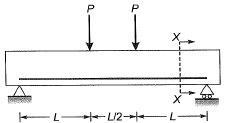At cross-section XX, which of the following statements is TRUE at the limit state?    [2015 : 1 Mark, Set-I]
(a) The variation of stress is linear and that of strain is non-linear
(b) The variation of strain is linear and that of stress is non-linear
(c) The variation of both stress and strain is linear
(d) The variation of both stress and strain is nonlinear
Ans.
(C)
Solution: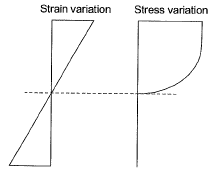Q.14 Workability of concrete can be measured using slump, compaction factor and Vee-bee time. Consider the following statements for workability of concrete
(i) As the slump increases, the Vee-bee time increases.
(ii) As the slump increases, the compaction factor increases.

Which of the following is TRUE?    [2015 : 1 Mark, Set-I]
(a) Both (i) and (ii) are True
(b) Both (i) and (ii) are False
(c) (i) is True and (ii) is False
(d) (i) is False and (ii) is True
Ans.
(D)
Solution:
As slump increase, water content increases means workability increases.
Compaction factor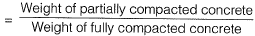∴ Compaction factor will also increase
The time required for complete remoulding in seconds is considered as a measure of workability and is expressed as the number of Vee-Beeseconds
∴ As workability increases, Vee-Bee time decreases.

Q.15 In a reinforced concrete section, the stress at the extreme fibre In compression is 5,80 MPa. The depth of neutral axis in the section is 58 mm and the grade of concrete is M25. Assuming linear elastic behavior of the concrete, the effective curvature of the section (in per mm) is    [2014 : 2 Marks, Set-I]
(a) 2.0 x 10-6
(b) 3.0 x 10-6
(c) 4.0 x 10-6
(d) 5.0 x 10-6
Ans.
(C)
Solution: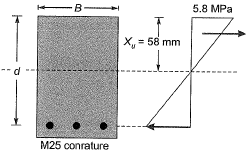Modulus of elasticity of concrete,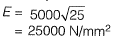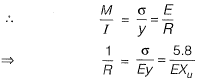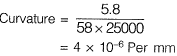⇒ Curvature = 4 x 10-6 per mm

Q.16 The flexural tensile strength of M25 grade of concrete, in N/mm2, as per IS 456 : 2000 is ____.    [2014 : 1 Mark, Set-II]
Solution:

Flexural strength =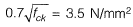Q.17 Group-I contains representative stress-strain curves as shown in the figure, while Group-I I gives the list of materials. Match the stress-strain curves with the corresponding materials.    [2014 : 1 Mark, Set-II]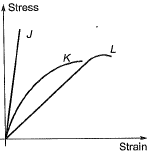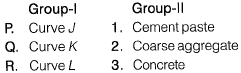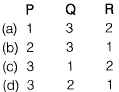Ans.
(B)
Solution:
The learner or less stiller the material, lesser is the slope of stress-strain curve, which is a measure of modulus of elasticity. Hence the diagram is self explanatory.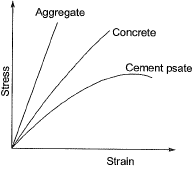Q.18 While designing, for a steel column of Fe250 grade, a base plate resting on a concrete pedestal of M20 grade, the bearing strength of concrete (in N/mm2) in limit state method of design as per IS 456 : 2000 i s _______ .    [2014 : 1 Mark, Set-I]
Solution:

Permissible bearing stress = 0.45 fck
= 0.45 x 20 = 9 N/mm2

Q.19 Maximum possible value of compaction factor for fresh (green) concrete is    [2013 : 1 Mark]
(a) 0.5
(b) 1.0
(c) 1.5
(d) 2.0
Ans.
(B)
Solution:
Compaction factor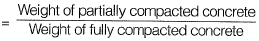Hence, its maximum value for fresh concrete can be 1.

Q.20 As per IS 456: 2000, in the Limit State Design of a flexural member, the strain in reinforcing bars under tension at ultimate state should not be less than    [2012 : 1 Mark]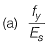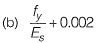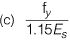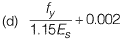Ans.
(D)
Solution: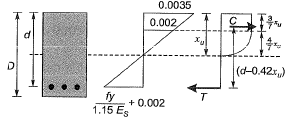Q.21 The cross-section of a thermo-mechanically treated (TMT) reinforcing bar has    [2011 : 1 Mark]
(a) soft ferrite-pearlite throughout.
(b) hard martensite throughout.
(c) a soft ferrite-pearlite core with a hard martensitic rim.
(d) a hard martensitic core with a soft pearlite- bainitic rim.
Ans.
(C)
Solution:
TMT or thermo mechanicaly treated bars are high strength reinforcement bars having a tough outer core and a soft inner core.
They provide strength is well as enough ductility to the structure.

Q.22 Match List-I (List of test methods for evaluating properties of concrete) with List-ll (List of properties) and select the correct answer using the codes given below the lists:    [2010 : 2 Marks]
List-I
A. Resonant frequency test
B. Rebound hammer test
C. Split cylinder test
D. Compacting factor test
List-ll
1. Tensile strength
2. Dynamic modulus of elasticity
3. Workability
4. Compressive strength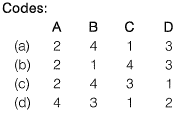Ans.
(A)
Solution:

A. Resonant frequency test is used to determine dynamic modulus of elasticity whereas destructive test like cube test etc measures the static modulus of elasticity.
B. Rebound hammer test is a non-destructive test used for compressive strength of concrete.
C. Split cylinder test is used to determine the direct tensile strength of concrete.
D. Compaction factor test is used to measure workability of concrete.

Offer running on EduRev: Apply code STAYHOME200 to get INR 200 off on our premium plan EduRev Infinity!

## RCC & Prestressed Concrete

14 videos|24 docs|12 tests

,

,

,

,

,

,

,

,

,

,

,

,

,

,

,

,

,

,

,

,

,

;